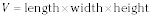##### Geometry: 1,001 Practice Problems For Dummies (+ Free Online Practice)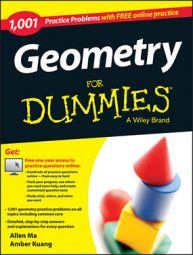Geometry is full of formulas, properties, and theorems. You can become successful in geometry by remembering the most important ones and learning how to apply them. Use this reference sheet as you practice various geometry problems to grow your knowledge and skills.

## Geometry practice problems with triangles and polygons

A polygon is a geometric figure that has at least three sides. The triangle is the most basic polygon. You will find the following formulas and properties useful when answering questions involving triangle inequalities, right triangles, relationships between the angles and sides of triangles, and interior and exterior angles of polygons.

## All triangles

• The sum of the three interior angles of a triangle is 180°.

• The largest side of a triangle is opposite the largest angle of the triangle.

• The sum of the two shorter sides of a triangle must be greater than the longest side of the triangle.

• The exterior angle of a triangle is equal to the sum of the two nonadjacent interior angles of the triangle.

• The centroid of a triangle divides each median of the triangle into segments with a 2:1 ratio.

## Right triangles

• The Pythagorean theorem states that a2 + b2 = c2, where a and b represent the legs of the right triangle and c represents the hypotenuse.

• When you draw an altitude to the hypotenuse of a right triangle, you form two right triangles that are similar to each other and also similar to the original right triangle. Because these triangles are similar, you can set up the following proportions:

• The altitude to the hypotenuse of a right triangle is the mean proportional between the two segments that the hypotenuse is divided into: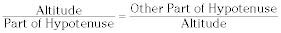• The leg of a right triangle is the mean proportional between the hypotenuse and the projection of the leg on the hypotenuse: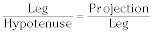•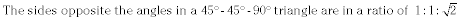•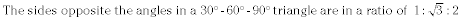• Here are the trigonometric ratios in a right triangle: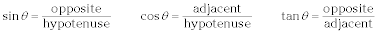## Polygons

• The sum of the degree measure of the interior angles of a polygon equals 180(n – 2), where n represents the number of sides.

• The sum of the exterior angles of a polygon is 360°.

• The area of a regular polygon equals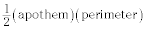The apothem is the line segment from the center of the polygon to the midpoint of one of the sides.

## Coordinate geometry formulas

When you work in geometry, you sometimes work with graphs, which means you’re working with coordinate geometry. Becoming familiar with the formulas and principles of geometric graphs makes sense, and you can use the following formulas and concepts as you graph: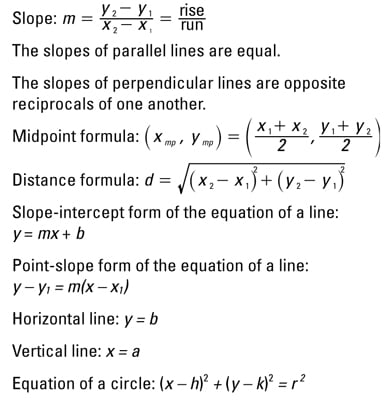## Transformation rules

In coordinate geometry problems, there are special rules for certain types of transformations. To determine the image point when performing reflections, rotations, translations and dilations, use the following rules:

• Reflections: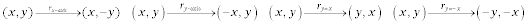• Rotations: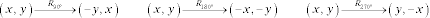• Translations: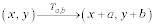• Dilations: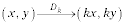## Circle basics for geometry problems

To solve geometry problems about circles, you will need to know the following circle theorems involving tangents, secants, and chords. These theorems can be used to find information about angles, intercepted arcs, and length of segments of a circle. In addition, you find the standard and general form of a circle, the formulas for area and circumference, and the area of a sector of a circle.

## Circle formulas

• The circumference of a circle equals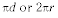• The area of a circle equals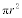• The area of a sector equals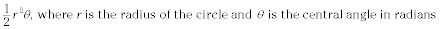•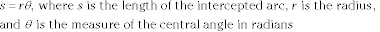• Standard form of a circle: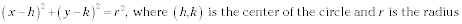• General form of a circle: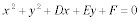## Circle theorems involving angles

• The central angle equals the intercepted arc.

• An inscribed angle equals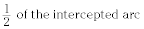• The interior vertical angles formed by two intersecting chords equal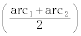• An exterior angle equals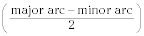• A line tangent to a circle is perpendicular to the radius drawn to the point of tangency.

## Circle theorems involving lengths of segments

• When a tangent and secant are drawn from the same exterior point,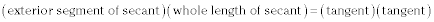• When two secants are drawn from the same exterior point,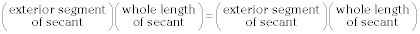## Formulas for geometric solids problems

Many formulas are associated with the study of three-dimensional shapes in geometry. Here, you find formulas for calculating the volume, surface area, and lateral area of cylinders, cones, spheres, pyramids, cube, and rectangular prisms.

## Cylinders

• The lateral area of a cylinder equals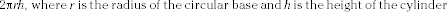•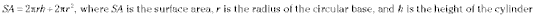•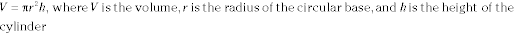## Cones

• The lateral area equals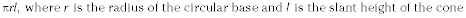•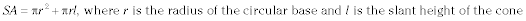•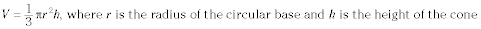## Spheres

•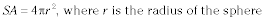•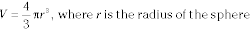## Square pyramids

•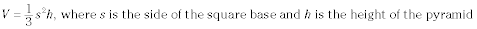## Cubes

•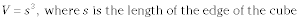## Rectangular prisms

•